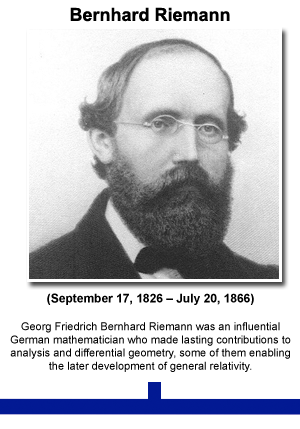Conformally Euclidean geometry of the upper half-plane

In Deriving invariant hyperbolic Riemannian metric on the half-plane we have calculated the line element for the SL(2,R) invariant hyperbolic geometry on the upper half-plane:

(1)Using this formula we can easily calculate the length of any segment of any curve. Ifis a parametric equation of the curve, thenand so

(2)(3)In this last formula we have used the fact that in the upper half-plane we haveFrom the studies of geometry of surfaces and its generalization developed mainly by Riemann we know that the formula for the line element allows us not only to find the length, but also the angles.

From Wikipedia:Bernhard Riemann – see Modern Science Map
Riemannian geometry originated with the vision of Bernhard Riemann expressed in his inaugural lecture “Ueber die Hypothesen, welche der Geometrie zu Grunde liegen” (“On the Hypotheses on which Geometry is Based”). It is a very broad and abstract generalization of the differential geometry of surfaces in. Development of Riemannian geometry resulted in synthesis of diverse results concerning the geometry of surfaces and the behavior of geodesics on them, with techniques that can be applied to the study of differentiable manifolds of higher dimensions.

It allows us to calculate scalar products of tangent vectors. Let us rewrite Eq. (1) in the form:using the symmetric (i.e.) “Riemannian metric” matrixThus, from Eq. (1), we haveand. Or

(4)In general, ifandare two tangent vectors atthen their scalar product is given by a general formula

(5)which in our case specializes to

(6)The numerator is exactly the same as for the Euclidean scalar product. It has the consequence that the angles in the hyperbolic upper half-plane geometry are the same as in the Euclidean geometry. That it is so follow from the definitions. Angles in any Riemannian geometry are defined the same way as in the Euclidean geometry. Ifare two tangent vectors atthen the anglebetween them is defined through the formula:

(7)whereandare their norms. If we use the scalar product given by Eq. (6), then the denominatorincancels out with the product of denominators is the norms. The end result is

(8).
Therefore, when we look at the picture like the one obtained in the last post:Esher’s Limit Circle IV developed onto upper half-plane. Click on the image to open it in full resolution.

all hyperbolic geometry angles between various lines at any particular joining point are the same as the perceived ones by our eyes ones – contrary to the sizes, where SL(2,R) invariant geometry and Euclidean geometry we are used to are different.

Whenever we have two metrics,andthat differ a scalar factorwe say that they are conformally related, or conformal to each other. In our case the metricin Eq. (4) is proportional to the identity matrix, our geometry is conformally Euclidean.

In the next note we will start calculating “straight lines”, or “geodesics” of our geometry. Some of them are almost evident candidates: vertical lines, perpendicular to the real line. But what about the other ones?

Our geometry is a toy geometry, as simple as possible. The group S(1,1) (or SL(2,R) ) is too simple. But one step further there is SU(2,2) – which became the basis for the “chronometric cosmology” developed by the late mathematician Irving Ezra Segal.

… The number of astronomers convinced was small, with experimentalists providing a slightly more favorable reception than theoreticians. Occasionally, the controversy was quite theatrical. A younger colleague of Segal was assaulted on Massachusetts Avenue by an astrophysicist still fuming from a Segalian conversation, and the publication of all the correspondence to and from Cambridge would be delightful. Particularly vituperative referee reports were always posted on his office door, some years overflowing onto the walls. Tee-shirts emblazoned with “Save Energy—Stop the Expansion of the Universe” can still be seen in Harvard Square. Whatever the final judgment is on the chronometric theory, Segal’s contributions to mathematics and mathematical physics have already had profound influence. Segal will be remembered for his conviction that the proper role of mathematics is to illuminate physics and his constant, irascible charm. We will also remember the perfect host, pouring wine for many a dinner, discussing physics, mathematics, philosophy, the proper preparation of coffee, and life.